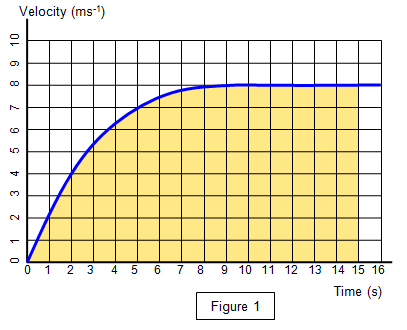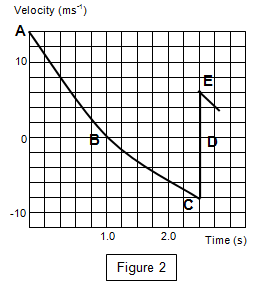# Non-uniform accelerated motion

In this case there are no simple equations we can use at this level. If the equation of the motion is known then calculations can be carried out.

However problems of non-uniform acceleration can be solved by using graphical methods. One example is the 100m sprint shown below. (Figure 1)### The 100 m sprint

This example of non-uniform acceleration provides a useful practical example of velocity time graphs.

The graph in Figure 1 shows how the velocity of a schoolgirl sprinter varies with time during a 100 m race. Notice that she accelerates rapidly at the start, the graph is steep, and then reaches a constant velocity after about 8s. The shaded area represents a distance of 100m and so she took 15 s to complete the race.

The gradient of the line at any point gives the instantaneous acceleration at that point.

Example problem – non-uniform accelerationA light rubber ball is thrown vertically upwards at 14 ms-1 and Figure 2 represents the velocity-time graph for part of the resulting motion.
(a) Describe the motion briefly.
(b) Estimate the acceleration at A, and the accelerations at B and C. How do you account for the differences between them?
(c) Estimate the height to which the ball rises above the point of projection

(a) The ball is thrown upwards and decelerates with a decreasing acceleration until it reaches the top at B. It then descends to the bottom at C when it bounces.
(b) The accelerations at A, B and C are found by taking the gradient of the curve at those points.
Acceleration at A = -17.5 ms-2
Acceleration at B = -10.0 ms-2
Acceleration at C = - 3.3 ms-2
(c) Since the acceleration is not uniform we cannot use the simple equations of motion to calculate the distance travelled; the area under the curve must be used instead.
Using this method the height to which the ball rises = 6.3 m (area AOB)

A VERSION IN WORD IS AVAILABLE ON THE SCHOOLPHYSICS USB

© Keith Gibbs 2020